## Difference Between Absolute and Relative Cell Referencing

In Excel we often reference another cell or a range of cells in a function or formula. And we drag the formula down the row so we don’t type the same formula again and again. Inevitably there will be times we want Excel to lock in a particular cell or range and there will be times we want Excel to keep shifting the cell up or down or across as we drag the formula. This is where absolute and relative cell referencing are useful.

## Absolute Cell Referencing

• Absolute Cell Referencing is when we lock in a particular cell or range such that even when we drag the formula across to other cells, the cell or range referenced in the formula does not change.

### How Do We Do This?

We do this by adding “\$” signs in front of the referenced cell or range in the formula. As an example, we can lock in A1 in the formula below: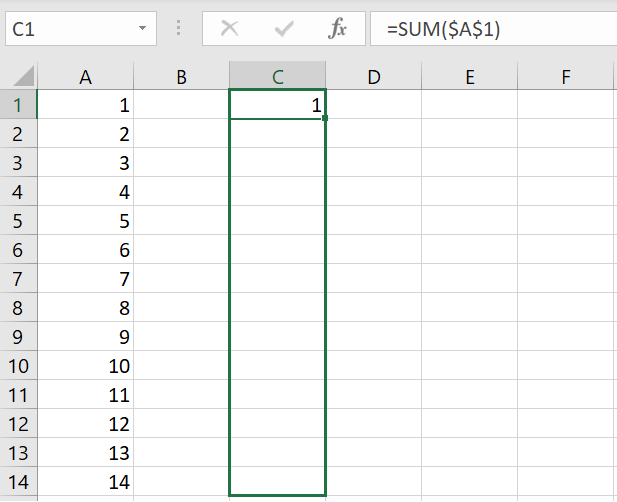By adding “\$” to A1, we lock in A1 so that when we drag the formula down, Excel will continue to calculate =SUM(\$A\$1). Without the “\$”, by default, Excel would shift the cell down so that C2 will be =SUM(A2), C3 will be =SUM(A3) and so on.

You can see above there are two “\$”s with \$A\$1. This is because you can specify whether you want to absolute reference the column or the row. In the example above, if we have \$A1 instead of \$A\$1, because Row 1 is not locked in, when we drag the formula down, Excel will shift the cell down to \$A2, \$A3, \$A4 and so on. And because the formulas are all in Column A, absolute referencing Column A in the formulas is actually not very useful at all.

## Relative Cell Referencing

Have you ever copied a cell because it has a certain value in it but when you paste it in another cell, the value is completely different in the pasted cell?

• Relative Cell Referencing is the opposite of absolute cell referencing and this is by default in Excel. It means when you first reference a cell or range in your current cell, Excel takes into account the location of that referenced cell or range in relation to the current cell you are typing in.

As mentioned this is the default in Excel. As an example below, if we reference A1 in C1, as we drag the formula down, Excel will automatically shift the referenced cell down by one each time. Such that in C2, the formula becomes =SUM(A2).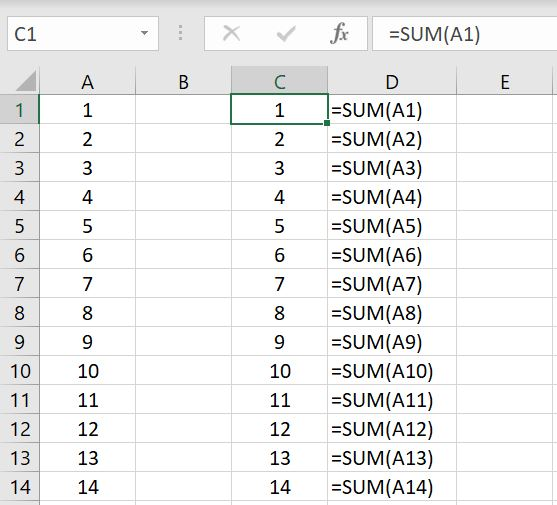This applies to more than dragging formulas. As an example below, we put “123” in Cell A1 and in Cell C3, we put “=A1”.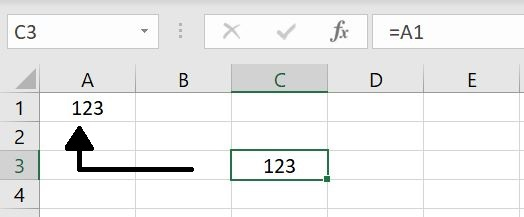By doing this, Excel has recognized that we are reference a cell that is “two cells to its left and two cells above”. If we now copy and paste Cell C3 and paste in Cell E5, Excel will recognize that we want to reference a cell that is “two cells to its left and two cells above” – in this case C3.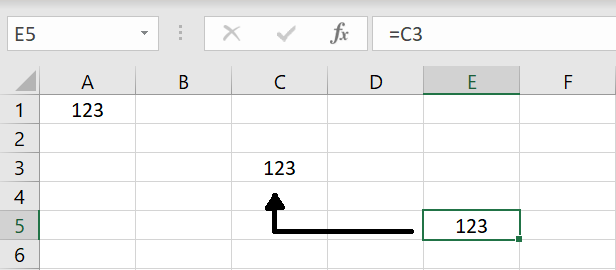Of course we need to keep in mind this is not restricted to what we’ve put in A1. If we now put in “Andrew” in another random cell, say Cell B6, and we copy Cell C3 or Cell E5 and paste it in Cell D8, Cell D8 will still pick up whatever is in Cell B6 because it is two cells to its left and two cells above:## Using Absolute and Relative Cell Referencing in the Same Formula

Of course we can use both absolute and relative cell referencing in the same formula in Excel. We do that a lot in COUNTIF function where we absolute reference the criteria_range and use relative referencing for the criteria. We will go through an example below. Here we have monthly revenues listed down and in Column D, we want to calculate year-to-date revenue. This means for January, the YTD revenue will just be January. For February, it will be January and February. For March, it’ll be January to March and so on: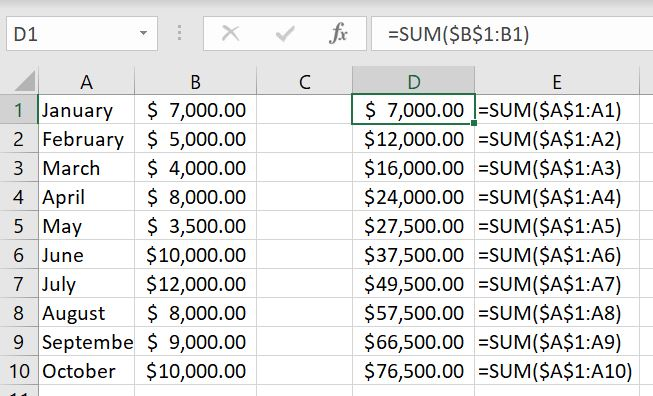In essence, we are calculating the total revenue from January to current month. This means we want to absolute reference January but relative reference current month because current month changes every month. By typing for January:

• =SUM(\$B\$1:B1)
• We are essentially just putting January revenue in D1. But as we drag the formula down, \$B\$1 remains as the first cell in the range but the second cell – which is relative referenced: B1 – will keep shifting down to B2, B3, B4 and so on accordingly each time.